\begin{equation}
\mathrm{ln}y=w^Tx+b
\end{equation}

# 目录

• Logistic 分布
• Logistic 函数
• 极大似然估计(MLE)
• Python代码实现及分析

# 1. Logistic 分布

\begin{equation}
F(x)=P(X\le{x})=\frac{1}{1+e^{-(x-\mu)/\gamma}}
\end{equation}

\begin{equation}
f(x)=F’(x)=\frac{e^{-(x-\mu)/\gamma}}{\gamma{(1+e^{-(x-\mu)/\gamma})}^2}
\end{equation}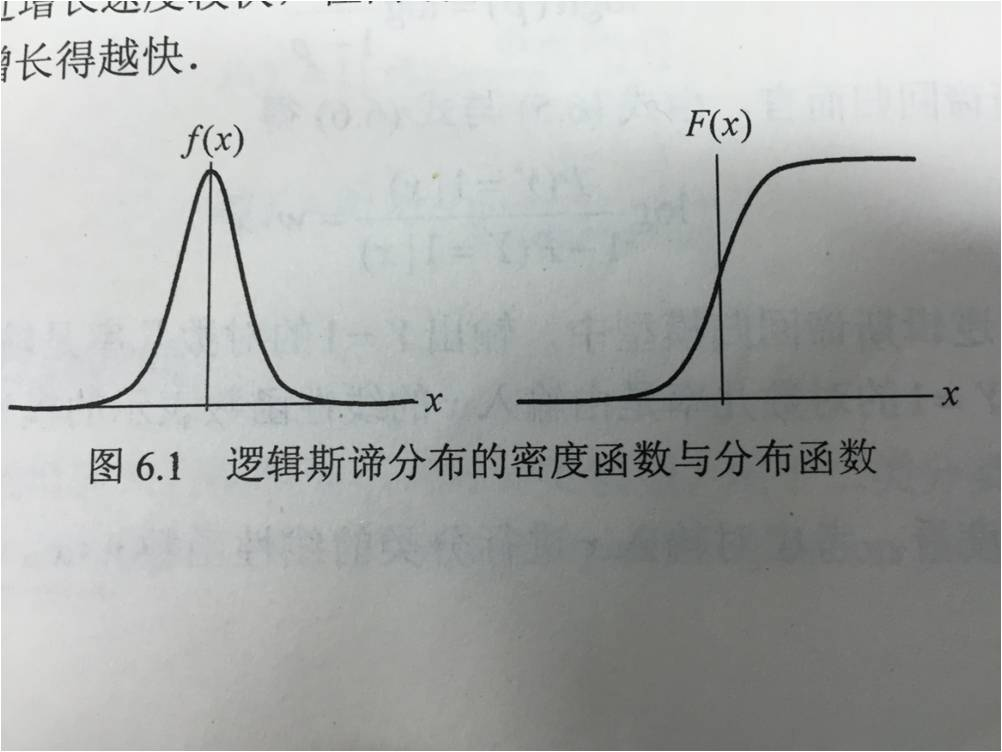# 2. Logistic 函数

\begin{equation}
y=\frac{1}{1+e^{-z}}
\end{equation}

logistic 函数是一种“Sigmoid函数”，它将$z$值转化为一个接近 0 或 1 的$y$值，并且输出值在$z=0$附近的变化很陡峭，若预测值大于零就判为正例，小于零就判为反例，预测值为临界值零则可任意判别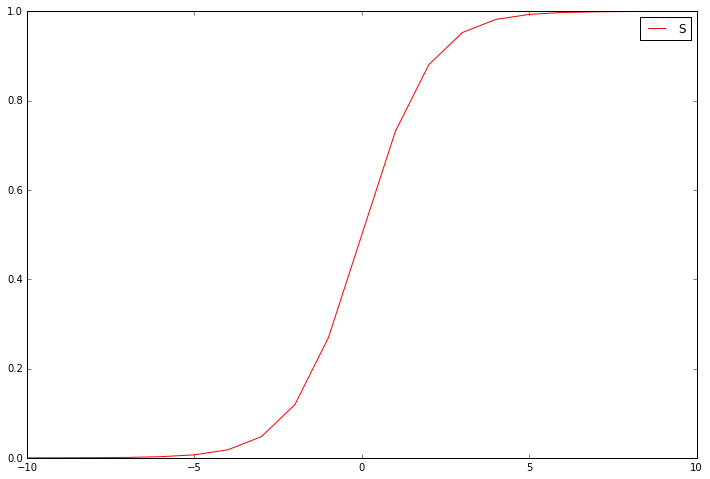\begin{equation}
y=\frac{1}{1+e^{-(w^Tx+b)}}
\end{equation}

\begin{equation}
\mathrm{ln}\frac{y}{1-y}=w^Tx+b
\label{zheng}
\end{equation}

# 3. 极大似然估计(MLE)\begin{equation}
h_w(x)=g(w^Tx)=\frac{1}{1+e^{-w^Tx}}
\end{equation}

\begin{equation}
\begin{aligned}
p(y=1|x)&=h_w(x)\
p(y=0|x)&=1-h_w(x)\
p(y|x)&={h_w(x)}^y{(1-h_w(x))}^{1-y}, y=0, 1
\end{aligned}
\end{equation}

\begin{equation}
\begin{aligned}
L(w)&=\prod{i=1}^{m}p(y^{(i)}|x^{(i)};\theta) \
&=\prod
{i=1}^{m}h_w{(x^{(i)})}^{y^{(i)}}{(1-h_w(x^{(i)}))}^{1-y^{(i)}}
\end{aligned}
\end{equation}

\begin{equation}
logL(w)=\sum_{i=1}^{m}[y^{(i)}logh_w(x^{(i)})+(1-y^{(i)})log(1-h_w(x^{(i)}))]
\end{equation}

\begin{equation}
J(w)=-\frac{1}{m}\sum{i=1}^{m}[y^{(i)}logh_w(x^{(i)})+(1-y^{(i)})log(1-h_w(x^{(i)}))]\
J(w)=-\frac{1}{m}\sum
{i=1}^{m}[y^{(i)}log\frac{hw(x^{(i)})}{1-h_w(x^{(i)})}+log(1-h_w(x^{(i)}))]\
J(w)=-\frac{1}{m}\sum
{i=1}^{m}[y^{(i)}(w^Tx)-log(1+e^{w^Tx})]
\end{equation}

\begin{equation}
{\theta}_j:={\theta}_j-\alpha\frac{\partial}{\partial{\theta}_j}J(\theta)
\end{equation}

\begin{equation}\begin{split}
\frac{\partial}{\partial{w}j}J(w) =& \frac{\partial{J(w)}}{\partial{h_w(x)}} \cdot \frac{\partial{h_w(x)}}{\partial{w}_j} \
=& [y^{(i)}+(y^{(i)}-1)/(1-h_w(x^{(i)})]\cdot [h_w(x^{(i)})(1-h_w(x^{(i)}))] x^{(i)}\
=& [(y^{(i)}h_w(x^{(i)})(1-h_w(x^{(i)}))+h_w(x^{(i)}(1-y^{(i)}))]\cdot x^{(i)}\
=& (h
{w}(x^{(i)})-y^{(i)})\cdot x_j^{(i)}
\end{split}\end{equation}

# 5. Python 代码实现 LR 及分析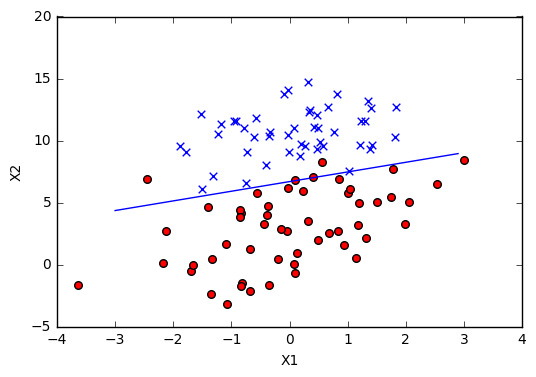# 6. 继续优化：随机梯度下降（SGD）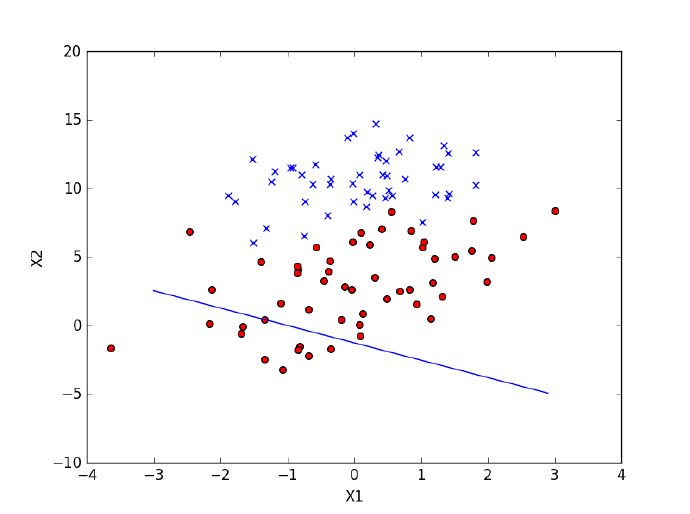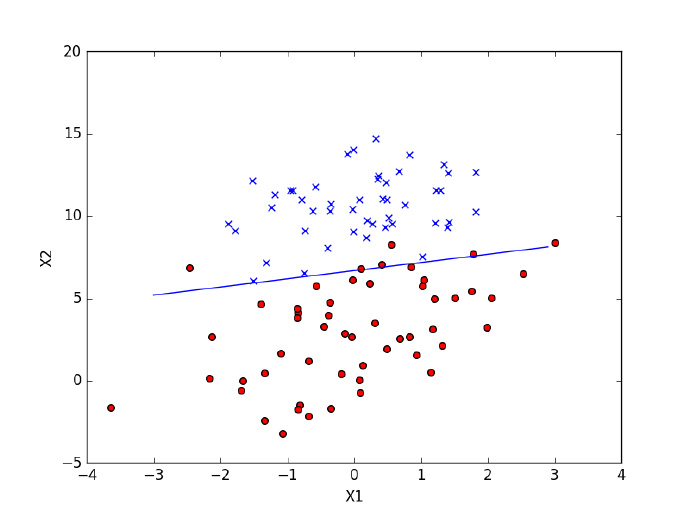# 关于 Logistic Regression 的讨论

1. 为什么 LR 模型要使用 sigmoid 函数？

1. 逻辑斯蒂回归？

Logistic Regression 中文翻译为“逻辑斯谛回归”，梳理了一下周志华老师在微博上的叙述，整理如下：Logistic Regression 与中文的“逻辑”没有半点关系，不是 logic，而是 logit，logistic 大概的意思是“logit 的”，而不是“log 的”，所以周老师将 Logistic Regression 翻译为对数几率回归。感兴趣的同学可以继续阅读这篇文章：趣谈“logistic”：物流？后勤？还是“逻辑斯谛”？

1. 逻辑回归如何进行多分类？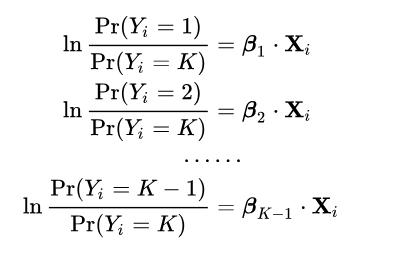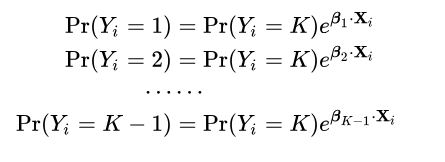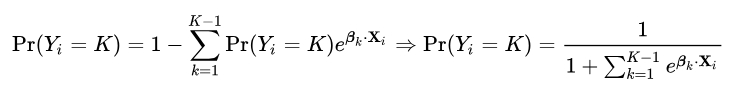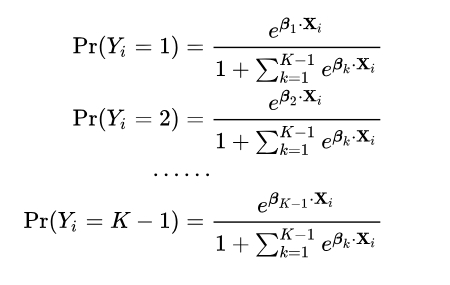# 推荐阅读

------本文结束，欢迎收藏本站、分享、评论或联系作者！------

(>看完记得五星好评哦亲<)
0%